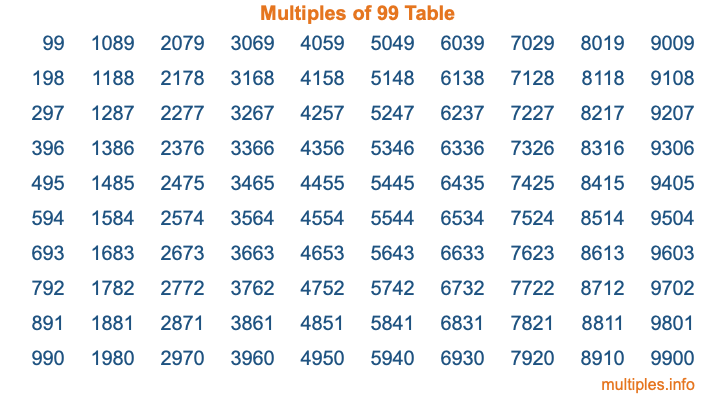Multiples of 99Welcome to the Multiples of 99 page. Here we will first teach you everything you will ever need to know about the multiples of 99, and then give you a study guide summary of everything we taught you to make sure you remember it all. Use this page to look up facts and learn information about the multiples of 99. This page will make you a multiples of ninety-nine expert!

Definition of Multiples of 99
Multiples of 99 are all the numbers that when divided by 99 equal an integer. Each of the multiples of 99 are called a multiple. A multiple of 99 is created by multiplying 99 by an integer.

Therefore, to create a list of multiples of 99, you start with 1 multiplied by 99, then 2 multiplied by 99, then 3 multiplied by 99, and so on for as long as you want. Thus, the list of the first five multiples of 99 is 99, 198, 297, 396, and 495. To see a larger list of multiples of 99, see the printable image of Multiples of 99 further down on this page. We also have a category where you can choose any nth multiple of 99.

Multiples of 99 Checker
The Multiples of 99 Checker below checks to see if any number of your choice is a multiple of 99. In other words, it checks to see if there is any number (integer) that when multiplied by 99 will equal your number. To do that, we divide your number by 99. If the the quotient is an integer, then your number is a multiple of 99.

Is  a multiple of 99?

Least Common Multiple of 99 and ...
A Least Common Multiple (LCM) is the lowest multiple that two or more numbers have in common. This is also called the smallest common multiple or lowest common multiple and is useful to know when you are adding our subtracting fractions. Enter one or more numbers below (99 is already entered) to find the LCM.

Check out our LCM Calculator if you need more details about the Least Common Multiple or if you need the LCM for different numbers for adding and subtraction fractions.

nth Multiple of 99
As we stated above, 99 is the first multiple of 99, 198 is the second multiple of 99, 297 is the third multiple of 99, and so on. Enter a number below to find the nth multiple of 99.

th multiple of 99

Multiples of 99 vs Factors of 99
99 is a multiple of 99 and a factor of 99, but that is where the similarities end. All postive multiples of 99 are 99 or greater than 99. All positive factors of 99 are 99 or less than 99.

Below is the beginning list of multiples of 99 and the factors of 99 so you can compare:

Multiples of 99: 99, 198, 297, 396, 495, etc.

Factors of 99: 1, 3, 9, 11, 33, 99

As you can see, the multiples of 99 are all the numbers that you can divide by 99 to get a whole number. The factors of 99, on the other hand, are all the whole numbers that you can multiply by another whole number to get 99.

It's also interesting to note that if a number (x) is a factor of 99, then 99 will also be a multiple of that number (x).

Multiples of 99 vs Divisors of 99
The divisors of 99 are all the integers that 99 can be divided by evenly. Below is a list of the divisors of 99.

Divisors of 99: 1, 3, 9, 11, 33, 99

The interesting thing to note here is that if you take any multiple of 99 and divide it by a divisor of 99, you will see that the quotient is an integer.

Multiples of 99 Table
Below is an image of the first 100 multiples of 99 in a table. The table is in chronological order, column by column. The first column has the first ten multiples of 99, the second column has the next ten multiples of 99, and so on.The Multiples of 99 Table is also referred to as the 99 Times Table or Times Table of 99. You are welcome to print out our table for your studies.

Negative Multiples of 99
Although not often discussed or needed in math, it is worth mentioning that you can make a list of negative multiples of 99 by multiplying 99 by -1, then by -2, then by -3, and so on, to get the following list of negative multiples of 99:

-99, -198, -297, -396, -495, etc.

Multiples of 99 Summary
Below is a summary of important Multiples of 99 facts that we have discussed on this page. To retain the knowledge on this page, we recommend that you read through the summary and explain to yourself or a study partner why they hold true.

There are an infinite number of multiples of 99.

A multiple of 99 divided by 99 will equal a whole number.

99 divided by a factor of 99 equals a divisor of 99.

The nth multiple of 99 is n times 99.

The largest factor of 99 is equal to the first positive multiple of 99.

99 is a multiple of every factor of 99.

99 is a multiple of 99.

A multiple of 99 divided by a divisor of 99 equals an integer.

99 divided by a divisor of 99 equals a factor of 99.

Any integer times 99 will equal a multiple of 99.

Multiples of a Number
Here you can get the multiples of another number, all with the same attention to detail as we did for multiples of 99 on this page.

Multiples of
Multiples of 100
Did you find our page about multiples of ninety-nine educational? Do you want more knowledge? Check out the multiples of the next number on our list!